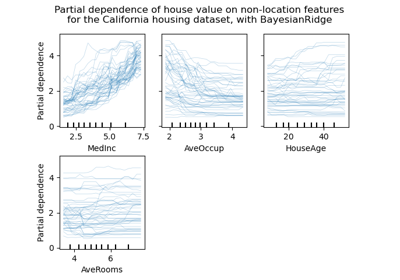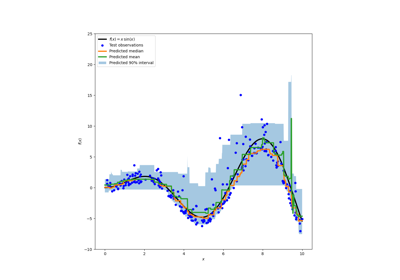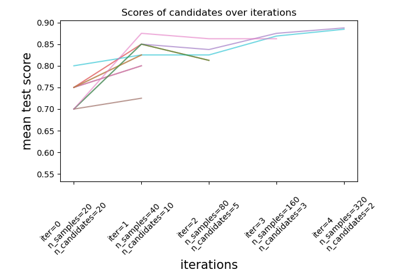# sklearn.model_selection.HalvingRandomSearchCV¶

class sklearn.model_selection.HalvingRandomSearchCV(estimator, param_distributions, *, n_candidates='exhaust', factor=3, resource='n_samples', max_resources='auto', min_resources='smallest', aggressive_elimination=False, cv=5, scoring=None, refit=True, error_score=nan, return_train_score=True, random_state=None, n_jobs=None, verbose=0)[source]

Randomized search on hyper parameters.

The search strategy starts evaluating all the candidates with a small amount of resources and iteratively selects the best candidates, using more and more resources.

The candidates are sampled at random from the parameter space and the number of sampled candidates is determined by n_candidates.

Read more in the User guide.

Note

This estimator is still experimental for now: the predictions and the API might change without any deprecation cycle. To use it, you need to explicitly import enable_halving_search_cv:

>>> # explicitly require this experimental feature
>>> from sklearn.experimental import enable_halving_search_cv # noqa
>>> # now you can import normally from model_selection
>>> from sklearn.model_selection import HalvingRandomSearchCV

Parameters:
estimatorestimator object

This is assumed to implement the scikit-learn estimator interface. Either estimator needs to provide a score function, or scoring must be passed.

param_distributionsdict

Dictionary with parameters names (string) as keys and distributions or lists of parameters to try. Distributions must provide a rvs method for sampling (such as those from scipy.stats.distributions). If a list is given, it is sampled uniformly.

n_candidatesint, default=’exhaust’

The number of candidate parameters to sample, at the first iteration. Using ‘exhaust’ will sample enough candidates so that the last iteration uses as many resources as possible, based on min_resources, max_resources and factor. In this case, min_resources cannot be ‘exhaust’.

factorint or float, default=3

The ‘halving’ parameter, which determines the proportion of candidates that are selected for each subsequent iteration. For example, factor=3 means that only one third of the candidates are selected.

resource'n_samples' or str, default=’n_samples’

Defines the resource that increases with each iteration. By default, the resource is the number of samples. It can also be set to any parameter of the base estimator that accepts positive integer values, e.g. ‘n_iterations’ or ‘n_estimators’ for a gradient boosting estimator. In this case max_resources cannot be ‘auto’ and must be set explicitly.

max_resourcesint, default=’auto’

The maximum number of resources that any candidate is allowed to use for a given iteration. By default, this is set n_samples when resource='n_samples' (default), else an error is raised.

min_resources{‘exhaust’, ‘smallest’} or int, default=’smallest’

The minimum amount of resource that any candidate is allowed to use for a given iteration. Equivalently, this defines the amount of resources r0 that are allocated for each candidate at the first iteration.

• ‘smallest’ is a heuristic that sets r0 to a small value:

• n_splits * 2 when resource='n_samples' for a regression problem

• n_classes * n_splits * 2 when resource='n_samples' for a classification problem

• 1 when resource != 'n_samples'

• ‘exhaust’ will set r0 such that the last iteration uses as much resources as possible. Namely, the last iteration will use the highest value smaller than max_resources that is a multiple of both min_resources and factor. In general, using ‘exhaust’ leads to a more accurate estimator, but is slightly more time consuming. ‘exhaust’ isn’t available when n_candidates='exhaust'.

Note that the amount of resources used at each iteration is always a multiple of min_resources.

aggressive_eliminationbool, default=False

This is only relevant in cases where there isn’t enough resources to reduce the remaining candidates to at most factor after the last iteration. If True, then the search process will ‘replay’ the first iteration for as long as needed until the number of candidates is small enough. This is False by default, which means that the last iteration may evaluate more than factor candidates. See Aggressive elimination of candidates for more details.

cvint, cross-validation generator or an iterable, default=5

Determines the cross-validation splitting strategy. Possible inputs for cv are:

• integer, to specify the number of folds in a (Stratified)KFold,

• An iterable yielding (train, test) splits as arrays of indices.

For integer/None inputs, if the estimator is a classifier and y is either binary or multiclass, StratifiedKFold is used. In all other cases, KFold is used. These splitters are instantiated with shuffle=False so the splits will be the same across calls.

Refer User Guide for the various cross-validation strategies that can be used here.

Note

Due to implementation details, the folds produced by cv must be the same across multiple calls to cv.split(). For built-in scikit-learn iterators, this can be achieved by deactivating shuffling (shuffle=False), or by setting the cv’s random_state parameter to an integer.

scoringstr, callable, or None, default=None

A single string (see The scoring parameter: defining model evaluation rules) or a callable (see Defining your scoring strategy from metric functions) to evaluate the predictions on the test set. If None, the estimator’s score method is used.

refitbool, default=True

If True, refit an estimator using the best found parameters on the whole dataset.

The refitted estimator is made available at the best_estimator_ attribute and permits using predict directly on this HalvingRandomSearchCV instance.

error_score‘raise’ or numeric

Value to assign to the score if an error occurs in estimator fitting. If set to ‘raise’, the error is raised. If a numeric value is given, FitFailedWarning is raised. This parameter does not affect the refit step, which will always raise the error. Default is np.nan.

return_train_scorebool, default=False

If False, the cv_results_ attribute will not include training scores. Computing training scores is used to get insights on how different parameter settings impact the overfitting/underfitting trade-off. However computing the scores on the training set can be computationally expensive and is not strictly required to select the parameters that yield the best generalization performance.

random_stateint, RandomState instance or None, default=None

Pseudo random number generator state used for subsampling the dataset when resources != 'n_samples'. Also used for random uniform sampling from lists of possible values instead of scipy.stats distributions. Pass an int for reproducible output across multiple function calls. See Glossary.

n_jobsint or None, default=None

Number of jobs to run in parallel. None means 1 unless in a joblib.parallel_backend context. -1 means using all processors. See Glossary for more details.

verboseint

Controls the verbosity: the higher, the more messages.

Attributes:
n_resources_list of int

The amount of resources used at each iteration.

n_candidates_list of int

The number of candidate parameters that were evaluated at each iteration.

n_remaining_candidates_int

The number of candidate parameters that are left after the last iteration. It corresponds to ceil(n_candidates[-1] / factor)

max_resources_int

The maximum number of resources that any candidate is allowed to use for a given iteration. Note that since the number of resources used at each iteration must be a multiple of min_resources_, the actual number of resources used at the last iteration may be smaller than max_resources_.

min_resources_int

The amount of resources that are allocated for each candidate at the first iteration.

n_iterations_int

The actual number of iterations that were run. This is equal to n_required_iterations_ if aggressive_elimination is True. Else, this is equal to min(n_possible_iterations_, n_required_iterations_).

n_possible_iterations_int

The number of iterations that are possible starting with min_resources_ resources and without exceeding max_resources_.

n_required_iterations_int

The number of iterations that are required to end up with less than factor candidates at the last iteration, starting with min_resources_ resources. This will be smaller than n_possible_iterations_ when there isn’t enough resources.

A dict with keys as column headers and values as columns, that can be imported into a pandas DataFrame. It contains lots of information for analysing the results of a search. Please refer to the User guide for details.

best_estimator_estimator or dict

Estimator that was chosen by the search, i.e. estimator which gave highest score (or smallest loss if specified) on the left out data. Not available if refit=False.

best_score_float

Mean cross-validated score of the best_estimator.

best_params_dict

Parameter setting that gave the best results on the hold out data.

best_index_int

The index (of the cv_results_ arrays) which corresponds to the best candidate parameter setting.

The dict at search.cv_results_['params'][search.best_index_] gives the parameter setting for the best model, that gives the highest mean score (search.best_score_).

scorer_function or a dict

Scorer function used on the held out data to choose the best parameters for the model.

n_splits_int

The number of cross-validation splits (folds/iterations).

refit_time_float

Seconds used for refitting the best model on the whole dataset.

This is present only if refit is not False.

multimetric_bool

Whether or not the scorers compute several metrics.

classes_ndarray of shape (n_classes,)

Class labels.

n_features_in_int

Number of features seen during fit.

feature_names_in_ndarray of shape (n_features_in_,)

Names of features seen during fit. Only defined if best_estimator_ is defined (see the documentation for the refit parameter for more details) and that best_estimator_ exposes feature_names_in_ when fit.

New in version 1.0.

HalvingGridSearchCV

Search over a grid of parameters using successive halving.

Notes

The parameters selected are those that maximize the score of the held-out data, according to the scoring parameter.

All parameter combinations scored with a NaN will share the lowest rank.

Examples

>>> from sklearn.datasets import load_iris
>>> from sklearn.ensemble import RandomForestClassifier
>>> from sklearn.experimental import enable_halving_search_cv  # noqa
>>> from sklearn.model_selection import HalvingRandomSearchCV
>>> from scipy.stats import randint
>>> import numpy as np
...
>>> clf = RandomForestClassifier(random_state=0)
>>> np.random.seed(0)
...
>>> param_distributions = {"max_depth": [3, None],
...                        "min_samples_split": randint(2, 11)}
>>> search = HalvingRandomSearchCV(clf, param_distributions,
...                                resource='n_estimators',
...                                max_resources=10,
...                                random_state=0).fit(X, y)
>>> search.best_params_
{'max_depth': None, 'min_samples_split': 10, 'n_estimators': 9}


Methods

 Call decision_function on the estimator with the best found parameters. fit(X[, y, groups]) Run fit with all sets of parameters. get_params([deep]) Get parameters for this estimator. Call inverse_transform on the estimator with the best found params. Call predict on the estimator with the best found parameters. Call predict_log_proba on the estimator with the best found parameters. Call predict_proba on the estimator with the best found parameters. score(X[, y]) Return the score on the given data, if the estimator has been refit. Call score_samples on the estimator with the best found parameters. set_params(**params) Set the parameters of this estimator. Call transform on the estimator with the best found parameters.
property classes_

Class labels.

Only available when refit=True and the estimator is a classifier.

decision_function(X)[source]

Call decision_function on the estimator with the best found parameters.

Only available if refit=True and the underlying estimator supports decision_function.

Parameters:
Xindexable, length n_samples

Must fulfill the input assumptions of the underlying estimator.

Returns:
y_scorendarray of shape (n_samples,) or (n_samples, n_classes) or (n_samples, n_classes * (n_classes-1) / 2)

Result of the decision function for X based on the estimator with the best found parameters.

fit(X, y=None, groups=None, **fit_params)[source]

Run fit with all sets of parameters.

Parameters:
Xarray-like, shape (n_samples, n_features)

Training vector, where n_samples is the number of samples and n_features is the number of features.

yarray-like, shape (n_samples,) or (n_samples, n_output), optional

Target relative to X for classification or regression; None for unsupervised learning.

groupsarray-like of shape (n_samples,), default=None

Group labels for the samples used while splitting the dataset into train/test set. Only used in conjunction with a “Group” cv instance (e.g., GroupKFold).

**fit_paramsdict of string -> object

Parameters passed to the fit method of the estimator.

Returns:
selfobject

Instance of fitted estimator.

get_params(deep=True)[source]

Get parameters for this estimator.

Parameters:
deepbool, default=True

If True, will return the parameters for this estimator and contained subobjects that are estimators.

Returns:
paramsdict

Parameter names mapped to their values.

inverse_transform(Xt)[source]

Call inverse_transform on the estimator with the best found params.

Only available if the underlying estimator implements inverse_transform and refit=True.

Parameters:
Xtindexable, length n_samples

Must fulfill the input assumptions of the underlying estimator.

Returns:
X{ndarray, sparse matrix} of shape (n_samples, n_features)

Result of the inverse_transform function for Xt based on the estimator with the best found parameters.

property n_features_in_

Number of features seen during fit.

Only available when refit=True.

predict(X)[source]

Call predict on the estimator with the best found parameters.

Only available if refit=True and the underlying estimator supports predict.

Parameters:
Xindexable, length n_samples

Must fulfill the input assumptions of the underlying estimator.

Returns:
y_predndarray of shape (n_samples,)

The predicted labels or values for X based on the estimator with the best found parameters.

predict_log_proba(X)[source]

Call predict_log_proba on the estimator with the best found parameters.

Only available if refit=True and the underlying estimator supports predict_log_proba.

Parameters:
Xindexable, length n_samples

Must fulfill the input assumptions of the underlying estimator.

Returns:
y_predndarray of shape (n_samples,) or (n_samples, n_classes)

Predicted class log-probabilities for X based on the estimator with the best found parameters. The order of the classes corresponds to that in the fitted attribute classes_.

predict_proba(X)[source]

Call predict_proba on the estimator with the best found parameters.

Only available if refit=True and the underlying estimator supports predict_proba.

Parameters:
Xindexable, length n_samples

Must fulfill the input assumptions of the underlying estimator.

Returns:
y_predndarray of shape (n_samples,) or (n_samples, n_classes)

Predicted class probabilities for X based on the estimator with the best found parameters. The order of the classes corresponds to that in the fitted attribute classes_.

score(X, y=None)[source]

Return the score on the given data, if the estimator has been refit.

This uses the score defined by scoring where provided, and the best_estimator_.score method otherwise.

Parameters:
Xarray-like of shape (n_samples, n_features)

Input data, where n_samples is the number of samples and n_features is the number of features.

yarray-like of shape (n_samples, n_output) or (n_samples,), default=None

Target relative to X for classification or regression; None for unsupervised learning.

Returns:
scorefloat

The score defined by scoring if provided, and the best_estimator_.score method otherwise.

score_samples(X)[source]

Call score_samples on the estimator with the best found parameters.

Only available if refit=True and the underlying estimator supports score_samples.

New in version 0.24.

Parameters:
Xiterable

Data to predict on. Must fulfill input requirements of the underlying estimator.

Returns:
y_scorendarray of shape (n_samples,)

The best_estimator_.score_samples method.

set_params(**params)[source]

Set the parameters of this estimator.

The method works on simple estimators as well as on nested objects (such as Pipeline). The latter have parameters of the form <component>__<parameter> so that it’s possible to update each component of a nested object.

Parameters:
**paramsdict

Estimator parameters.

Returns:
selfestimator instance

Estimator instance.

transform(X)[source]

Call transform on the estimator with the best found parameters.

Only available if the underlying estimator supports transform and refit=True.

Parameters:
Xindexable, length n_samples

Must fulfill the input assumptions of the underlying estimator.

Returns:
Xt{ndarray, sparse matrix} of shape (n_samples, n_features)

X transformed in the new space based on the estimator with the best found parameters.

## Examples using sklearn.model_selection.HalvingRandomSearchCV¶Release Highlights for scikit-learn 0.24

Release Highlights for scikit-learn 0.24Prediction Intervals for Gradient Boosting Regression

Prediction Intervals for Gradient Boosting RegressionSuccessive Halving Iterations

Successive Halving Iterations